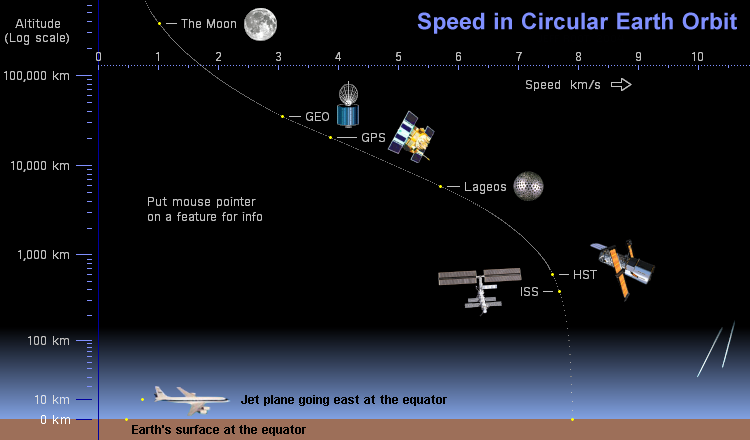ORBITAL SPEED

A satellite in orbit moves faster when it is close to the planet or other body that it orbits, and slower when it is farther away.  When a satellite falls from high altitude to lower altitude, it gains speed, and when it rises from low altitude to higher altitude, it loses speed.

A satellite in circular orbit has a constant speed which depends only on the mass of the planet and the distance between the satellite and the center of the planet.  Here are some examples of satellites in Earth orbit:Altitude r Speed Period Lifetime The Moon 385,000  km 391,370  km 1.01  km/s 27.3 days Billions of years 100,000  km 106,370  km 1.94  km/s 4 days Billions of years GEO 35,800  km 42,170  km 3.07  km/s 1 day Millions of years Navstar 20,200  km 26,570  km 3.87  km/s 12 hours Millions of years 10,000  km 16,370  km 4.93  km/s 5.8 hours Millions of years Lageos 5,900  km 12,270  km 5.70  km/s 3.8 hours Millions of years 2,000  km 8,370  km 6.90  km/s 2.1 hours Millenia 1,000  km 7,370  km 7.35  km/s 105 minutes Millenia Hubble 600  km 6,970  km 7.56  km/s 97 minutes Decades ISS 380  km 6,750  km 7.68  km/s 92 minutes Years 200  km 6,570  km 7.78  km/s 89 minutes Days or weeks 100  km 6,470  km 7.84  km/s 87 minutes Minutes Sea Level 0  km 6,370  km 7.90  km/s 84 minutes Seconds

The speed (v) of a satellite in circular orbit is:

v = SQRT(G * M / r)

where G is the universal gravitational constant (6.6726 E-11 N m2 kg-2), M is the mass of the combined planet/satellite system (Earth's mass is 5.972 E24 kg), and r is the radius of the orbit measured from the planet's center.  "SQRT" means "square root".

Using these values gives the speed in meters per second.

The period (P) of a satellite in circular orbit is the orbit's circumference divided by the satellite's speed:

P = 2 * pi * r / v

Using values in metric units, as above, gives the period in seconds.  (pi = 3.14159...)

Gravitational pulls from the Moon and Sun are the strongest forces perturbing orbits of Earth satellites above 40,000 km.

Off-center gravitational pull from Earth's equatorial bulge is the strongest force perturbing orbits of satellites between 500 km and 40,000 km.

Atmospheric drag is the strongest force perturbing orbits of Earth satellites below 500 km.  Dense satellites with small cross-sectional area are affected less than light satellites with large area.  A very dense satellite might just make a full orbit starting at 100 km.

Satellites in elliptical orbit move faster than the circular speed while near perigee, and slower than the circular speed while near apogee.  The period of a satellite in any orbit, circular or elliptical, is given by Kepler's third law:

P = 2 * pi * SQRT(r3 / G * M)

where r is the mean radius of the orbit -- that is, the apogee plus the perigee (measured from the planet's center) divided by two, or half the major axis of the ellipse.

Changes to the orbit of a satellite are most efficient at perigee and apogee.  A rocket burn at perigee which increases orbital speed raises the apogee.  A burn at perigee which decreases orbital speed lowers the apogee.  Likewise, a burn at apogee which increases orbital speed raises the perigee, and a burn at apogee which decreases orbital speed lowers the perigee.Atmospheric drag on a satellite at perigee lowers the apogee, causing the orbit to become more and more circular, until the entire orbit is at the perigee altitude, and the satellite soon falls from orbit.

The state of the atmosphere is also a factor.  Increasing activity in the eleven-year solar cycle heated Earth's upper atmosphere in the late 1970s, expanding it.  This increased the drag on Skylab, which was originally at 435 kilometers, and brought it down two years earlier than expected.

Changes in the inclination of a satellite's orbital plane are most efficient at apogee.  Large inclination changes require very large expenditures of fuel, so are rarely done.  A common plane change moves geosynchronous satellites to orbit directly over the equator.

Combining a change in altitude with a change in inclination is more efficient than using a separate engine burn for each.

Whether increasing or decreasing altitude or changing orbital inclination, the most efficient engine burns are parallel to the planet's surface.  When the Space Shuttle returned a large satellite to Earth, the de-orbit burn was deliberately made in an inefficient direction to use up extra fuel and lower the spacecraft's mass below the landing safety limit.

The most efficient way into orbit from Earth is to launch directly east from the equator.  This takes advantage of Earth's rotational speed, adding it to the speed provided by the launch vehicle.  The speed at the equator is 465 meters per second, roughly 6% of the speed needed to go into orbit.The S-shaped curve of orbital speeds in the graphic above is caused by the choice of origin for the logarithmic altitude scale.  The origin is 15 km below Earth's surface, to better show differences at low altitudes.  Putting it at Earth's center, to coincide with the mathematical equivalent of the origin of Earth's gravitational field, makes the curve simpler, with just one bend, as at right.

Jeff Root
September 18, 2004